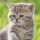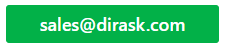Home
IT Knowledge
IT Career
Inspiration
Languages
EN

# Math - why cos(0) is equal 1?

1 points
Created by:Kate_C
19800

Explaination why `cos(0)=1` requires to refer to the function definition.

`cos(α)` has been defined as:

Lets suppose that: alpha angle goes to zero (`α -> 0`) and adjacent side is constant (`b = const`).

It causes: opposite side goes to zero too (`a -> 0`) and hypotenuse side goes to adjacent side (`h -> b`). When angle is equal to `0` and `b` is equal to `h` then `b` divided by `h` is equal to `1`. It means `cos(0)` is equal to `1` acording to above definition.

Simple visualisation is presented on below picture: